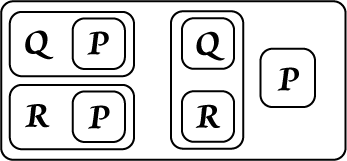Quantifier Distribution Principles

We will state these principles as implications and show corresponding Beta Graphs. Diagrams on the left were prepared in Adobe Illustrator; those on the right are done in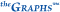.

1

(x)(Ax É Bx) É ((x)Ax É (x)Bx)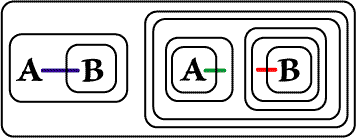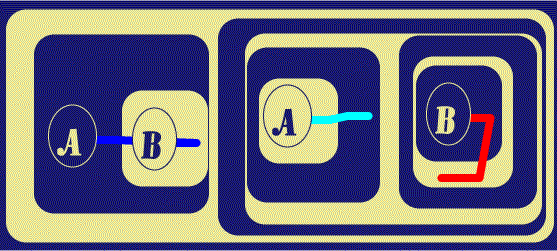2 (x)(Ax Ù Bx) É ((x)Ax Ù (x)Bx)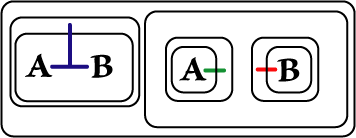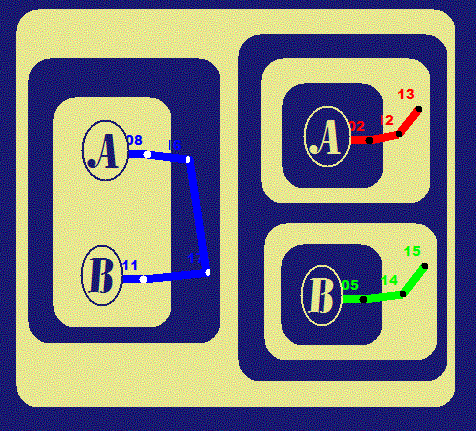3 ((x)Ax Ù (x)Bx) É (x)(Ax Ù Bx)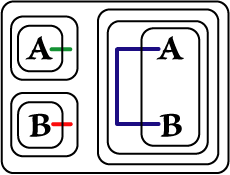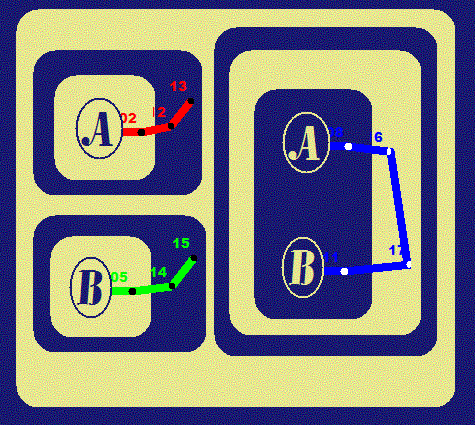4 ((\$x)Ax Ú (\$x)Bx) É (\$x)(Ax Ú Bx)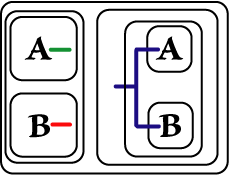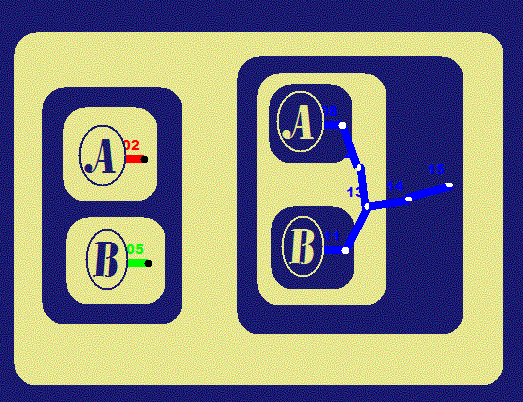5 (\$x)(Ax Ú Bx) É ((\$x)Ax Ú (\$x)Bx)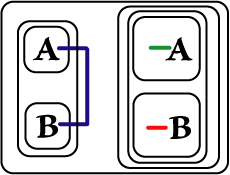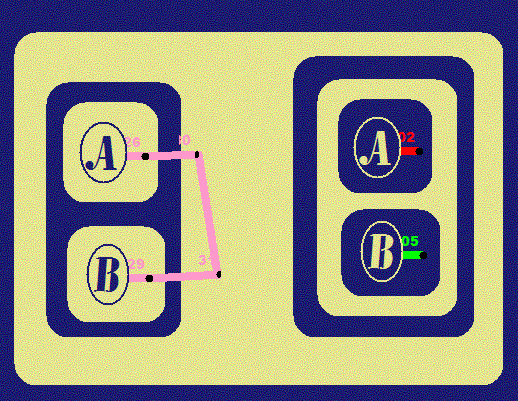6 ((x)Ax É (\$x)Bx) É (\$x)(Ax É Bx)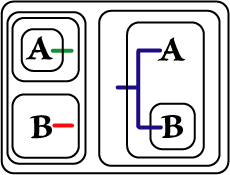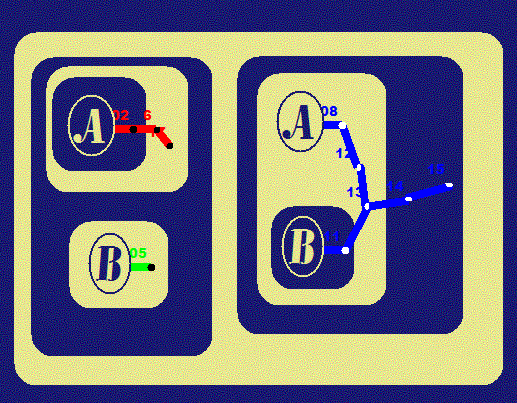7 (\$x)(Ax É Bx) É ((x)Ax É (\$x)Bx)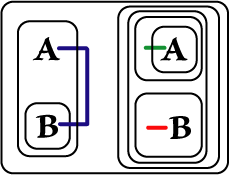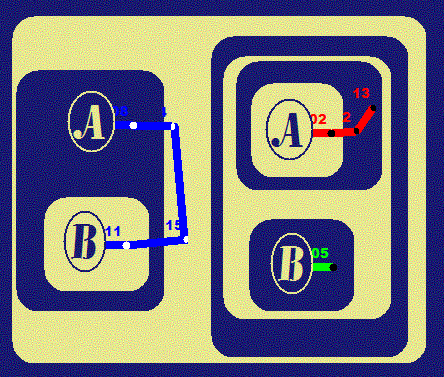8 ((\$x)Ax É (x)Bx) É (x)(Ax É Bx)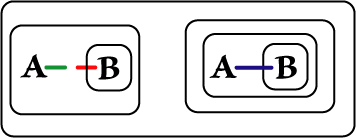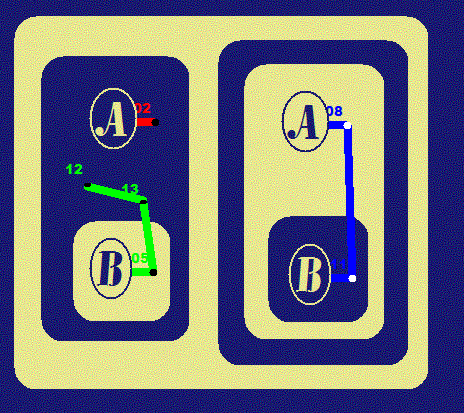Some Useful Alpha-provable Graphs

9 ((P É Q) Ù (P É R ) Ù P ) É (Q Ù R)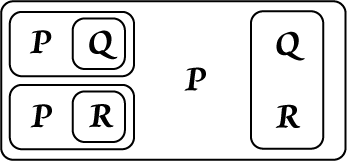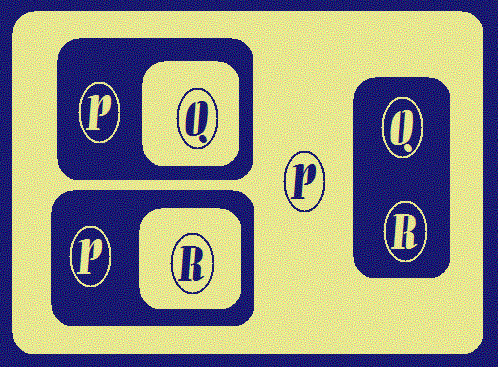10 ((Q É P) Ù (R É  P) Ù  (Q Ú R)) É P